## Tamilnadu Samacheer Kalvi 12th Maths Solutions Chapter 1 Applications of Matrices and Determinants Ex 1.7

Question 1.
Solve the following system of homogeneous equations.
(i) 3x + 2y + 7z = 0, 4x – 3y – 2z = 0, 5x + 9y + 23z = 0
(ii) 2x + 3y – z = 0, x – y – 2z = 0, 3x + y + 3z = 0
Solution:
(i) The matrix form of the above equations is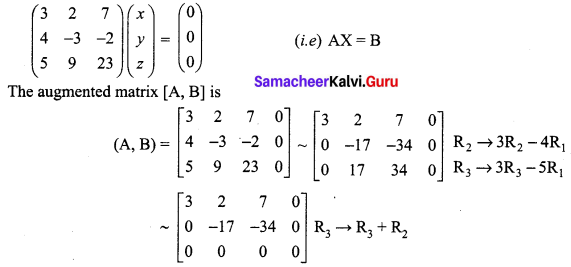The above matrix is in echelon form.
Here ρ(A, B) = ρ(A) < number of unknowns.
The system is consistent with infinite number of solutions. To find the solutions.
Writing the equivalent equations.
We get 3x + 2y + 7z = 0 ……. (1)
-17y – 34z = 0 ……. (2)
Taking z = t in (2) we get -17y – 34t = 0
⇒ -17y = 34t
⇒ y = -2t
Taking z = t, y = -2t in (1) we get
3x + 2(-2t) + 7t = 0
⇒ 3x – 4t + 7t = 0
⇒ 3x = -3t
⇒ x = -t
So the solution is x = -t; y = -2t; and z = t, t ∈ R
(ii) The matrix form of the equations is
$$\left(\begin{array}{ccc}{2} & {3} & {-1} \\ {1} & {-1} & {-2} \\ {3} & {1} & {3}\end{array}\right)\left(\begin{array}{l}{x} \\ {y} \\ {z}\end{array}\right)=\left(\begin{array}{l}{0} \\ {0} \\ {0}\end{array}\right)$$
(i.e) AX = B
The augmented matrix [A, B] is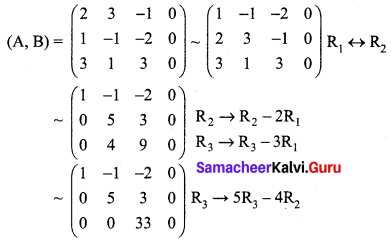The above matrix is in echelon form also ρ(A, B) = ρ(A) = 3 = number of unknowns
The system is consistent with unique solution, x = y = z = 0
(i.e) The system has trivial solution only.Question 2.
Determine the values of λ for which the following system of equations.
x + y + 3z = 0, 4x + 3y + λz = 0, 2x +y + 2z = 0 has
(i) a unique solution
(ii) a non-trivial solution
Solution:
The matrix form of the equation is
$$\left(\begin{array}{lll}{1} & {1} & {3} \\ {4} & {3} & {\lambda} \\ {2} & {1} & {2}\end{array}\right)\left(\begin{array}{l}{x} \\ {y} \\ {z}\end{array}\right)=\left(\begin{array}{l}{0} \\ {0} \\ {0}\end{array}\right)$$
(i.e) AX = B
The augmented matrix [A, B] is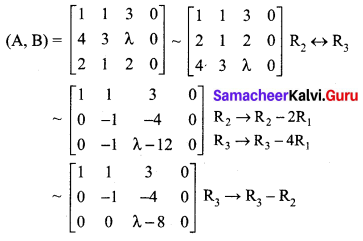The above matrix is in echelon form
Case 1: When λ ≠ 8, ρ(A, B) = ρ(A) = 3 = number of unknowns
The system is consistent with a unique solution.
Case 2: When λ = 8, ρ(A, B) = ρ(A) = 2 < number of unknowns.
The system is consistent with non-trivial solutions.

Question 3.
By using the Gaussian elimination method, balance the chemical reaction equation:
C2H6 + O2 → H2O + CO2
Solution:
We are searching for positive integers x1, x2, x3 and x4 such that
x1C2H6 + x2O2 = x3H2O + x4CO2 ……. (1)
The number of carbon atoms on LHS of (1) should be equal to the number of carbon atoms on the RHS of (1).
So we get a linear homogeneous equation.
2x1 = x4
⇒ 2x1 – x4 = 0 …… (2)
Similarly considering hydrogen and oxygen atoms we get respectively.
2x2 = x3 + 2x4
⇒ 2x2 – x3 – 2x4 = 0 …… (3)
and -2x3+ 3x4 = 0 …… (4)
Equations (2), (3) and (4) constitute a homogeneous system of linear equations in four unknowns.
The augmented matrix (A, B) is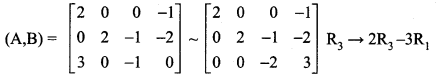Now ρ(A, B) = ρ(A) = 3 < number of unknowns.
So the system is consistent and has an infinite number of solutions.
Writing the equations using the echelon form we get
2x1 – x4 = 0 …… (5)
2x2 – x3 – 2x4 = 0 ……. (6)
-2x3 + 3x4 = 0 ……. (7)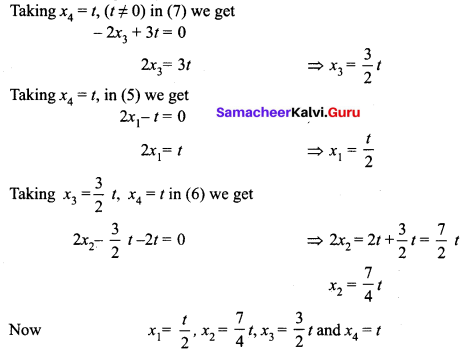Since x1, x2, x3 and x4 are positive integers. Let us choose t = 4t.
Then we get x1 = 2, x2 = 7, x3 = 6, and x4 = 4
So the balanced equation is 2C2H6 + 7O2 → 6H2O + 4CO2.### Samacheer Kalvi 12th Maths Solutions Chapter 1 Applications of Matrices and Determinants Ex 1.7 Additional Problems

Question 1.
Solve the following homogeneous linear equations.
x + 2y – 5z = 0,
3x + 4y + 6z = 0,
x + y + z = 0
Solution:
The given system of equations can be written in the form of matrix equation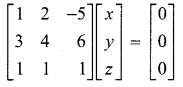AX = B
The augmented matrix is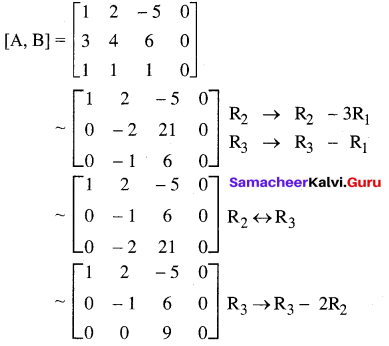This is in the echelon form.
Clearly ρ[A, B] = 3 and ρ(A) = 3
∴ ρ(A) = ρ[A, B] = 3 = number of unknowns
∴ The given system of equations is consistent and has a unique solution. i.e., trivial solution
∴ x = 0, y = 0 and z = 0
Note: Since ρ(A) = 3, | A | ≠ 0 i.e. A is non-singular;
∴ The given system has only trivial solution x = 0, y = 0, z = 0

Question 2.
For what value of n the equations.
x + y + 3z = 0,
4x + 3y + µz = 0,
2x + y + 2z = 0 have a
(i) trivial solution,
(ii) non-trivial solution.
Solution:
The system of equations can be written as AX = B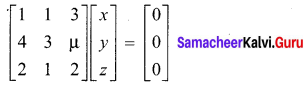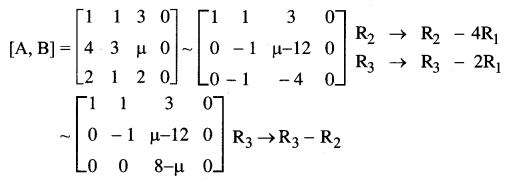Case (i): If µ ≠ 8 then 8 – µ ≠ 0 and hence there are three non-zero rows.
∴ ρ[A] = ρ[A, B] = 3 = the number of unknowns.
∴ The system has the trivial solution x = 0, y = 0, z = 0
Case (ii): If µ = 8 then.
ρ[A, B] = 2 and ρ(A) = 2
∴ ρ(A) = ρ[A, B] = 2 < number of unknowns.
The given system is equivalent to
x + y + 3z = 0; y + 4z = 0
∴ y= – 4z ; x = z
Taking z = k, we get x = k,y = – 4k, z = k [k ∈ R – {0}] which are non-trivial solutions.
Thus the system is consistent and has infinitely many non-trivial solutions.
Note: In case (ii) the system also has trivial solution. For only non-trivial solutions we removed k = 0.